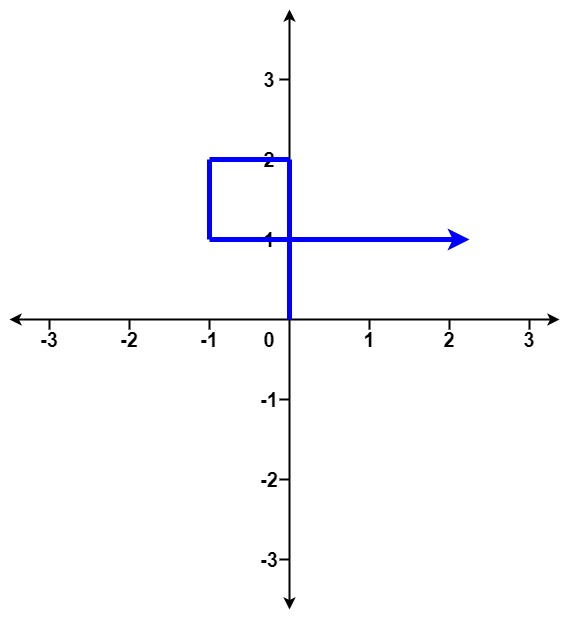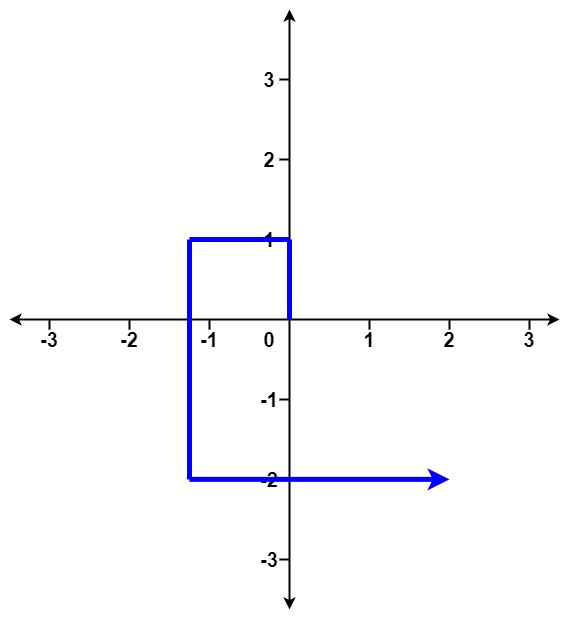| English | 简体中文 |

# 335. Self Crossing

## Description

You are given an array of integers distance.

You start at point (0,0) on an X-Y plane and you move distance meters to the north, then distance meters to the west, distance meters to the south, distance meters to the east, and so on. In other words, after each move, your direction changes counter-clockwise.

Return true if your path crosses itself, and false if it does not.

Example 1:Input: distance = [2,1,1,2]
Output: true


Example 2:Input: distance = [1,2,3,4]
Output: false


Example 3:Input: distance = [1,1,1,1]
Output: true


Constraints:

• 1 <= distance.length <= 105
• 1 <= distance[i] <= 105